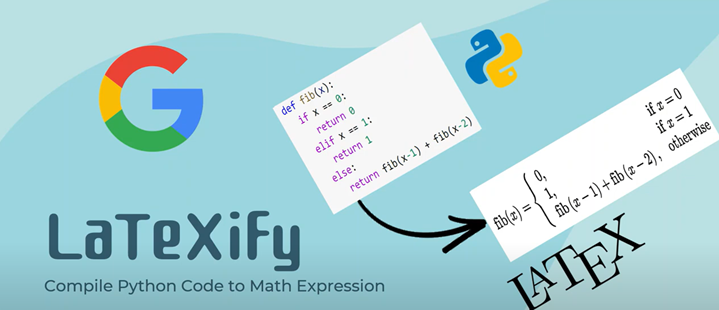# Statsmodels 统计包之OLS回归

Statsmodels和Scikit-learn是两个不同的Python库，它们都提供了用于线性回归的工具。Statsmodels中支持的线性回归模型列表：

• OLS回归：OLS代表“普通最小二乘回归”，它是一种最常见且最简单的线性回归模型。
• WLS回归：WLS代表“加权最小二乘回归”，它在处理异方差性（即误差项方差不相等）时比OLS回归更为有效。
• GLS回归：GLS代表“广义最小二乘回归”，它可以处理异方差性和相关性。
• GLSAR回归：GLSAR代表“广义最小二乘回归自回归误差模型”，它可以用于处理自相关误差。
• WLSIV回归：WLSIV代表“两阶段最小二乘回归”，它应用于当某些解释变量存在内生性时，可以采用这种回归方法来控制内生性。
• 交互作用回归：它可用于模拟两个或多个自变量之间的交互作用。
• 多项式回归：它添加了自变量的高次幂项以建立非线性关系。
• 岭回归：岭回归是一种正则化线性回归方法，旨在减少过拟合问题。
• Lasso回归：Lasso回归也是一种正则化线性回归方法，但与岭回归不同，它倾向于将一些系数缩小到零，从而实现特征选择。
• Elastic Net回归：Elastic Net回归是岭回归和Lasso回归的组合。

• API风格：statsmodels和Scikit-learn的API有所不同，这意味着在使用它们时需要编写不同的代码。例如，statsmodels的OLS使用类似R语言的formula语法，而Scikit-learn的线性回归则需要通过X和y参数传递数据。
• 推断统计：statsmodels的OLS回归提供了更多的统计信息，并可以执行各种假设检验、置信区间等推断统计操作。相反，Scikit-learn的线性回归主要专注于预测目标变量，其输出只包括系数和截距等基本信息。
• 数据处理：在数据预处理方面，Scikit-learn提供了许多常见的数据清洗和转换工具，例如填充缺失值、标准化数据等。在另一方面，statsmodels主要专注于对模型进行拟合和统计分析。
• 应用场景：由于针对的应用场景不同，建议根据实际需求选择合适的工具。如果主要关注的是推断统计，则建议使用statsmodels的OLS回归；如果主要关注预测目标变量，则建议使用Scikit-learn的线性回归模型。

## Statsmodels中OLS的使用

import pandas as pd
from statsmodels.formula.api import ols

model = ols("y ~ x", data=data).fit()

print(model.summary())


                            OLS Regression Results
==============================================================================
Dep. Variable:                      y   R-squared:                       0.568
Method:                 Least Squares   F-statistic:                     101.4
Date:                Wed, 08 Nov 2023   Prob (F-statistic):           1.07e-15
Time:                        13:58:37   Log-Likelihood:                 249.14
No. Observations:                  79   AIC:                            -494.3
Df Residuals:                      77   BIC:                            -489.5
Df Model:                           1
Covariance Type:            nonrobust
==============================================================================
coef    std err          t      P>|t|      [0.025      0.975]
------------------------------------------------------------------------------
Intercept      0.1589      0.004     45.367      0.000       0.152       0.166
x              0.0872      0.009     10.069      0.000       0.070       0.104
==============================================================================
Omnibus:                        2.226   Durbin-Watson:                   0.941
Prob(Omnibus):                  0.329   Jarque-Bera (JB):                1.549
Skew:                           0.296   Prob(JB):                        0.461
Kurtosis:                       3.348   Cond. No.                         8.44
==============================================================================

Notes:
 Standard Errors assume that the covariance matrix of the errors is correctly specified.


OLS Regression Results的解读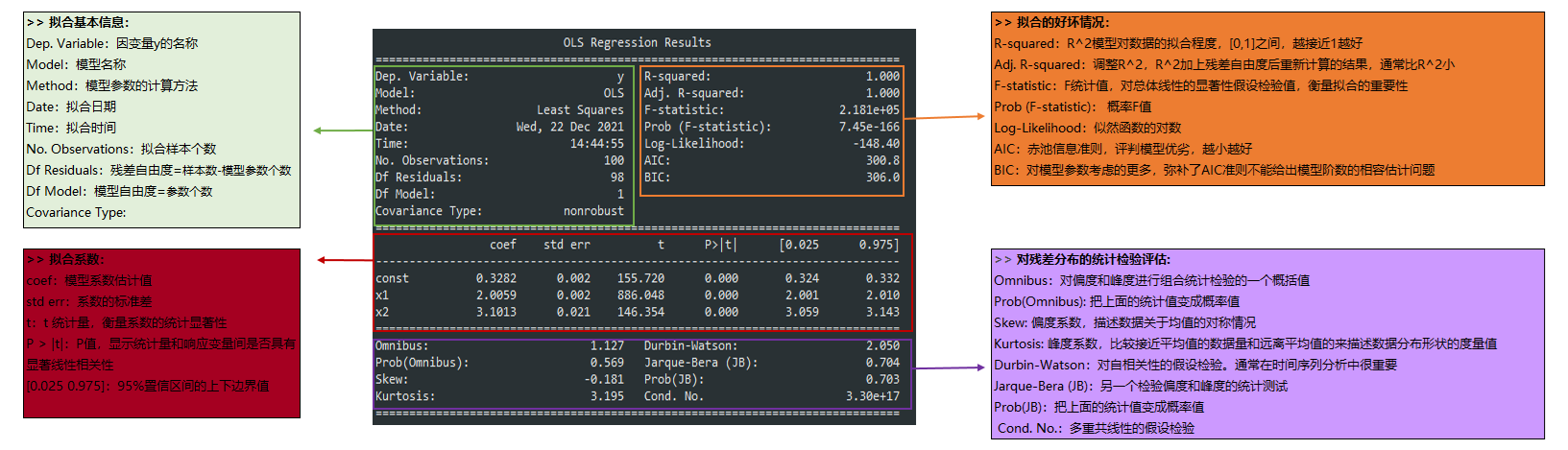Summary内容较多，其中重点考虑参数R-squared、Prob(F-statistic)以及P>|t| 的两个值，通过这4个参数就能判断的模型是否是线性显著的，同时知道显著的程度如何。

• R-squared：决定系数，其值=SSR/SST，SSR是Sum of Squares for Regression，SST是Sum of Squares for Total，这个值范围在[0, 1]，其值越接近1，说明回归效果越好。
• F-statistic：这就是我们经常用到的F检验，这个值越大越能推翻原假设，本例中其值为4，这个值过大，说明我们的模型是线性模型，原假设是“我们的模型不是线性模型”。 如果显著性水平为0.05，则当自由度为1时，F检验临界值分别为3.84。
• Prob (F-statistic)：这就是上面F-statistic的概率，这个值越小越能拒绝原假设，本例中为25e-08，该值非常小了，足以证明我们的模型是线性显著的。
• P>|t|：统计检验中的P值，这个值越小越能拒绝原假设。P值的临界值取决于显著性水平，通常在统计假设检验中使用05或0.01作为显著性水平。这意味着如果P值小于0.05（或0.01），我们可以在95%（或99%）的置信水平下拒绝原假设，并得出结论表明结果是显著的。

coef_df = pd.DataFrame({"params": model.params,   # 回归系数
"std err": model.bse,     # 回归系数标准差
"t": round(model.tvalues,3),       # 回归系数T值
"p-values": round(model.pvalues,3) # 回归系数P值
})

coef_df[['coef_0.025','coef_0.975']] = model.conf_int() # 回归系数置信区间 默认5%，括号中可填具体数字 比如0.05, 0.1
coef_df

 params std err t p-values coef_0.025 coef_0.975 Intercept 0.158931 0.003503 45.367 0.0 0.151955 0.165907 x 0.087192 0.008660 10.069 0.0 0.069948 0.104435

Statsmodels的plot_regress_exog函数来帮助我们理解我们的模型。根据一个回归因子绘制回归结果。在一个2*2的图中绘制了四幅图:”endog vs exog”，”残差vs exog”，”拟合vs exog”和”拟合+残差vs exog”

import matplotlib.pyplot as plt
import statsmodels.api as sm

fig = plt.figure(figsize=(15,8))
fig = sm.graphics.plot_regress_exog(model,"x",fig=fig)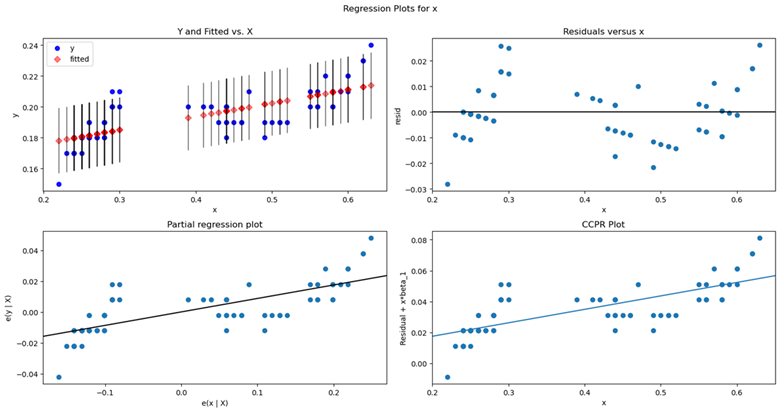from statsmodels.sandbox.regression.predstd import wls_prediction_std

# wls_prediction_std(model)返回三个值, 标准差，置信区间下限，置信区间上限
_, confidence_interval_lower, confidence_interval_upper = wls_prediction_std(model)

x = data['x']
y = data['y']
fig, ax = plt.subplots(figsize=(10,7))
ax.plot(x, y, 'bo', label="data")
ax.plot(x, model.fittedvalues, 'g--.', label="OLS")
ax.plot(x, confidence_interval_upper, 'r--')
ax.plot(x, confidence_interval_lower, 'r--')
ax.set_title('Simple Linear Regression')
ax.grid()
ax.legend(loc='best')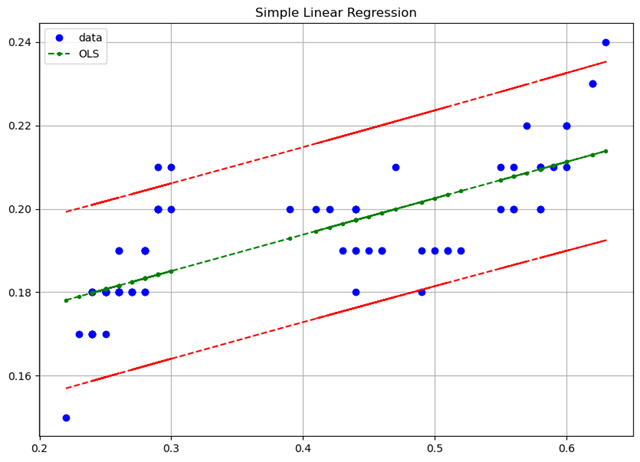# 多元线性模型构建
stock_models = ols("close ~ open + high + low + volume", data=data).fit()
# 用ols做非线性回归
lm_s = smf.ols(formula='y ~ np.sin(x)+x', data=df).fit()
# ridge
ols('y~x1+x2',data=df).fit_regularized(alpha=1, L1_wt=1)
# C指的是Categorical variables
res = smf.ols(formula='Lottery ~ Literacy + Wealth + C(Region)', data=df).fit()
# -1 指的是使 Intercept = 0
res = smf.ols(formula='Lottery ~ Literacy + Wealth + C(Region) -1 ', data=df).fit()
# “:” adds a new column to the design matrix with the product of the other two columns. “
# *” will also include the individual columns that were multiplied together:
# 所以'Lottery ~ Literacy * Wealth - 1' 相当于 'Lottery ~ Literacy + Wealth+Literacy : Wealth - 1'
res1 = smf.ols(formula='Lottery ~ Literacy : Wealth - 1', data=df).fit()
res2 = smf.ols(formula='Lottery ~ Literacy * Wealth - 1', data=df).fit()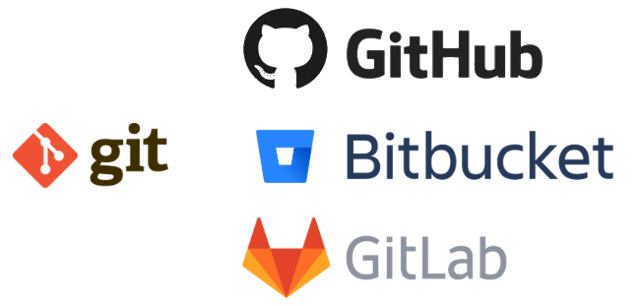##### GitHub非开发人员使用教程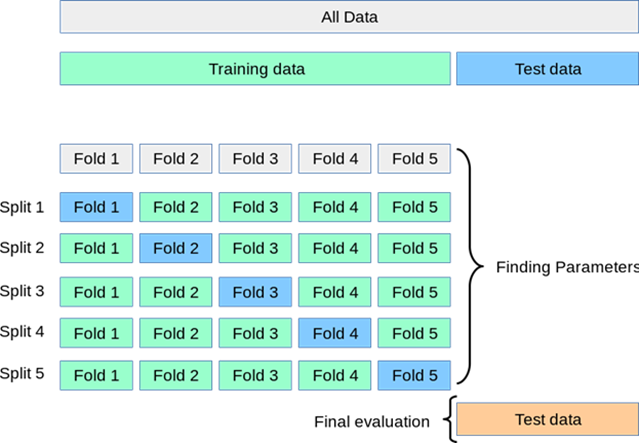##### Scikit-Learn学习之交叉验证